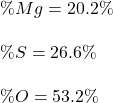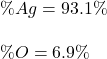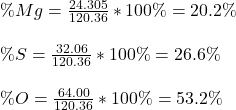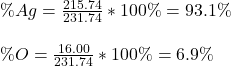## 2) Calculate the percent composition of each element in Mgso, 3) Calculate the percentage of each element in Ag,o.

Question

2) Calculate the percent composition of each element in Mgso,

3) Calculate the percentage of each element in Ag,o.

in progress 0
6 months 2021-07-11T13:35:23+00:00 1 Answers 7 views 0

2)3)Explanation:

Hello!

2) In this case, since magnesium sulfate is MgSO₄, we can see how magnesium weights 24.305 g/mol, sulfur 32.06 g/mol and oxygen 64.00 g/mol as there is one atom of magnesium as well as sulfur but four oxygen atoms for a total of g/mol; thus the percent compositions are:3) In this case, although the element seems to contain Ag and O, we infer its molecular formula is Ag₂O; thus, since we have two silver atoms weighing 215.74 g/mol and one oxygen atom weighing 16.00 g/mol for a total of 231.74 g/mol, we obtain the following percent compositions:Best regards!